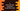# C program to check if a number is a disarium number or not## C program to check if a number is a disarium number or not:

In this post, we will learn how to find if a number is a disarium number or not in C programming language. The program will take a number as an input from the user, check if it is a disarium number or not and print a message.

Before we start to write down the program, let me quickly explain to you what is a disarium number the algorithm we will use.

### What is a Disarium number:

A number is called a disarium number if the sum of the digit of the number raised to the power of their positions is equal to the number itself. The position is calculated from left to right and it starts from 1. So, for the leftmost digit, it is 1, for second left digit, it is 2 etc.

For example, if abcd is the number, we need to find the value of a^1 + b^2 + c^3 + d^4 and if this is equal to the number, it is a disarium number. Else, it is not.

For example, 89 is a disarium number because the sum of 8^1 + 9^2 = 8 + 81 is 89 which is the number itself.

### Algorithm to find if a number is Disarium number or not:

It will be easy if we start from the rightmost digit instead of starting from the leftmost digit of a number.

We will use the below algorithm to find if a number is disarium number or not:

• Take the number as an input from the user.
• Make a copy of the number and store it in another variable.
• Find the length of the number on the copied number.
• Initialize a variable length as 0 to hold the length.
• By using a loop, keep removing the last digit of the number until it become 0. Also increment the value of length by 1 on each step. We will get the length of the number once the loop is completed.
• Since we are finding the length of the copied number, we can start on the original number to find the required sum.
• Initialize a number as 0 to hold the sum.
• By using a loop, we will pick the rightmost digits of the number and find the power of that digit raised to its position.
• Add that value to the sum variable and remove the rightmost digit.
• Once the loop is completed, compare the number with the sum value. If both are equal, it is a disarium number. Else, it is not a disarium number.

Let’s write down the program now.

### C program to check if a number is Disarium number or not:

Below is the complete program:

``````#include <stdio.h>
#include <math.h>

int findLength(int no)
{
int copiedNumber = no;
int length = 0;

while (copiedNumber != 0)
{
length++;
copiedNumber /= 10;
}

return length;
}

int isDisarium(int no, int length)
{
int sum = 0;
int copiedNumber = no;
int lastDigit;

while (copiedNumber > 0)
{
lastDigit = copiedNumber % 10;

sum += (int)pow(lastDigit, length);
copiedNumber /= 10;
length--;
}

return sum == no;
}

int main()
{
int no;
printf("Enter a number: ");

scanf("%d", &no);

int length = findLength(no);

if (isDisarium(no, length) == 1)
{
printf("%d is a Disarium number\n", no);
}
else
{
printf("%d is not a Disarium number\n", no);
}

return 0;
}``````

Here,

• findLength method is used to find the length of a number. It takes a number as its parameter, finds the length by using a loop and returns that. It makes a copy of a number and operates on that copy to find the length.
• isDisarium method is used to check if a number is a disarium number or not. It takes a number and the length of the number as the parameters and returns an integer. It returns 0 if the number is not disarium. Else it returns 1. This method also creates a copy of the number and works on that.
• The program takes a number as the input and stores it in the variable no.
• It finds the length by using the findLength method and stores that value in the length variable.
• It calls the isDisarium method and checks the return value. If it is 1, it prints that the number is a disarium number, else it prints that it is not a disarium number.

### Sample output:

If you run this program, it will print output as like below:

``````Enter a number: 175
175 is a Disarium number

Enter a number: 122
122 is not a Disarium number

Enter a number: 89
89 is a Disarium number``````